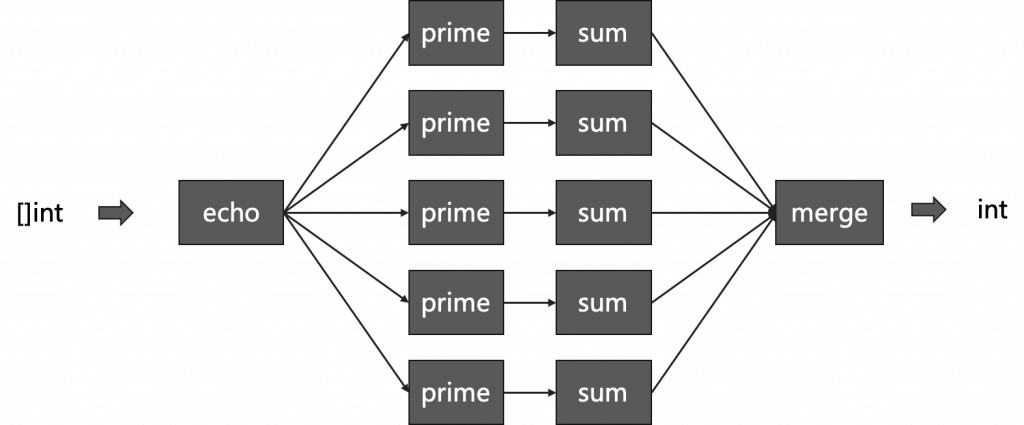Go编程模式：Pipeline

# Go编程模式：Pipeline### 本文是全系列中第8 / 10篇：Go编程模式

#### HTTP 处理

```http.HandleFunc("/v1/hello", WithServerHeader(WithAuthCookie(hello)))

```type HttpHandlerDecorator func(http.HandlerFunc) http.HandlerFunc
func Handler(h http.HandlerFunc, decors ...HttpHandlerDecorator) http.HandlerFunc {
for i := range decors {
d := decors[len(decors)-1-i] // iterate in reverse
h = d(h)
}
return h
}```

```http.HandleFunc("/v4/hello", Handler(hello,

#### Channel 管理

Rob Pike在 Go Concurrency Patterns: Pipelines and cancellation 这篇blog中介绍了如下的一种编程模式。

##### Channel转发函数

```func echo(nums []int) <-chan int {
out := make(chan int)
go func() {
for _, n := range nums {
out <- n
}
close(out)
}()
return out
}```

##### 平方函数
```func sq(in <-chan int) <-chan int {
out := make(chan int)
go func() {
for n := range in {
out <- n * n
}
close(out)
}()
return out
}
```
##### 过滤奇数函数
```func odd(in <-chan int) <-chan int {
out := make(chan int)
go func() {
for n := range in {
if n%2 != 0 {
out <- n
}
}
close(out)
}()
return out
}
```
##### 求和函数
```func sum(in <-chan int) <-chan int {
out := make(chan int)
go func() {
var sum = 0
for n := range in {
sum += n
}
out <- sum
close(out)
}()
return out
}```

```var nums = []int{1, 2, 3, 4, 5, 6, 7, 8, 9, 10}
for n := range sum(sq(odd(echo(nums)))) {
fmt.Println(n)
}```

```type EchoFunc func ([]int) (<- chan int)
type PipeFunc func (<- chan int) (<- chan int)

func pipeline(nums []int, echo EchoFunc, pipeFns ... PipeFunc) <- chan int {
ch  := echo(nums)
for i := range pipeFns {
ch = pipeFns[i](ch)
}
return ch
}```

```var nums = []int{1, 2, 3, 4, 5, 6, 7, 8, 9, 10}
for n := range pipeline(nums, gen, odd, sq, sum) {
fmt.Println(n)
}```

#### Fan in/Out

```func makeRange(min, max int) []int {
a := make([]int, max-min+1)
for i := range a {
a[i] = min + i
}
return a
}

func main() {
nums := makeRange(1, 10000)
in := echo(nums)

const nProcess = 5
var chans [nProcess]<-chan int
for i := range chans {
chans[i] = sum(prime(in))
}

for n := range sum(merge(chans[:])) {
fmt.Println(n)
}
}```

```func is_prime(value int) bool {
for i := 2; i <= int(math.Floor(float64(value) / 2)); i++ {
if value%i == 0 {
return false
}
}
return value > 1
}

func prime(in <-chan int) <-chan int {
out := make(chan int)
go func ()  {
for n := range in {
if is_prime(n) {
out <- n
}
}
close(out)
}()
return out
}```

• 我们先制造了从1到10000的一个数组，
• 然后，把这堆数组全部 `echo`到一个channel里 – `in`
• 此时，生成 5 个 Channel，然后都调用 `sum(prime(in))` ，于是每个Sum的Go Routine都会开始计算和
• 最后再把所有的结果再求和拼起来，得到最终的结果。

```func merge(cs []<-chan int) <-chan int {
var wg sync.WaitGroup
out := make(chan int)

for _, c := range cs {
go func(c <-chan int) {
for n := range c {
out <- n
}
wg.Done()
}(c)
}
go func() {
wg.Wait()
close(out)
}()
return out
}```#### 延伸阅读

（全文完）（转载本站文章请注明作者和出处 酷 壳 – CoolShell ，请勿用于任何商业用途）

——=== 访问 酷壳404页面 寻找遗失儿童。 ===——(37 人打了分，平均分： 4.00 )Loading...

## 《Go编程模式：Pipeline》的相关评论

1.tzongw说道：

fan in/out一节的例子，虽然中间分成5个channel和goroutine，但是最后又merge成一个channel。真正处理数据只会有一个goroutine，其余4个都是阻塞住的，没有并发起来。不知我理解的对不对？

1.说道：

也有此疑问。使用buffered channel可否提高并发性

1.陈皓说道：

merge就是需要待所有的channel都处理完成了。但是计算的事都并行完了，所以，在计算上是并发的，在merge上并不是。但真正耗时的是计算而不是merge，所以，并发是有用的。

另，使不使用buffered channel都差不多，无非就是阻塞在merge还是merge后而已。

2.网赚博客说道：

网赚博客：www.nizhuanqian.com

2.wardenlym说道：

same logic written in Elixir:

result =
input
|> prime
|> sum
|> merge

3.乌龟阿白说道：

耗子哥，可以打招聘广告么……不行您就删了哈

如果对耗子哥这几篇go编程模式都掌握的炉火纯青，热爱并熟悉函数式，热爱并熟悉函数式，热爱并熟悉函数式，又在寻找新的工作机会的同学。欢迎私聊 wx: starc_mo 坐标深圳，海外电商 SaaS 赛道，薪酬可与 BAT 竞争，寻找有野心的技术人入局。

1.Bourne说道：

不会是shopee吧，哈哈哈

4.绝了说道：

merge时是否使用buffered chan在这个demo里没多大区别。因为merge是将5个no buffered chan合并到一个no buffered chan，并最终使用sum消费这个chan。瓶颈在最后一步的sum操作。

5.商机文库说道：

介绍的很详细

6.bdws说道：

快递刷单单号购买www.chaojidanhao.cn

7.neohope说道：

第一次调用sum时启动了5个goroutine，然后在merge中等待5个goroutine全部完成。

第二次调用sum就没有用到并发了。而且第二次的goroutine编号，与第一次的没有任何重叠。

虽然是pipeline，其实每次调用都用了不同的goroutine，这和多线程编程是不一样的。

8.就是玩说道：

多goroutine 加int,不是线程安全的，需要使用atomic执行加法

9.dwqdwqd说道：

带我去的无群多

10.小超人说道：

请问is_prime中的实现，int(math.Floor(float64(value)/2))为什么要这么写啊？我自己验证发现效果和value/2一样。